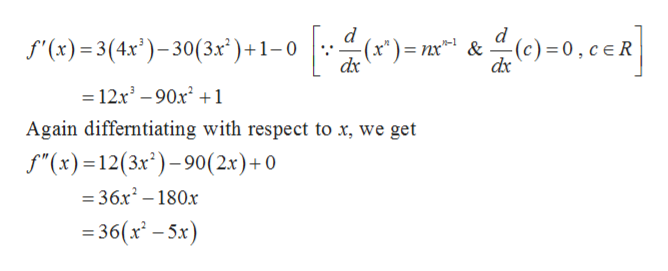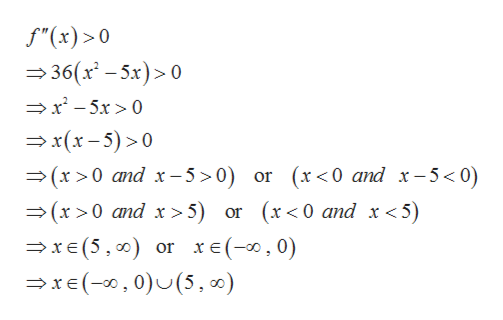# Determine where the graph of the function is concave upward and where it is concave downward. (Enter your answers using interval notation. If the answer cannot be expressed as an interval, enter EMPTY or ∅.)f(x) = 3x4 − 30x3 + x − 4

Question
106 views

Determine where the graph of the function is concave upward and where it is concave downward. (Enter your answers using interval notation. If the answer cannot be expressed as an interval, enter EMPTY or ∅.)

f(x) = 3x4 − 30x3 + x − 4
check_circle

Step 1

Given,

Step 2

The graph of y = f (x) is concave upward on those intervals where its second derivative is greater than zero and the graph of

y = f (x) is concave downward on those intervals where its second derivative is less than zero.

Therefore differentiating given function with respect to x, we gethelp_outlineImage Transcriptionclosed (x*)nx&(c) = 0, ceR dx f(x)3(4x)-30(3x')+1-0 12x3-90x1 Again differntiating with respect to x, we get f"(x)12(3x)-90(2x)+0 =36x2-180x 36(x-5x) fullscreen
Step 3

Now computing the values of x for which second deriva...help_outlineImage Transcriptionclosef"(x) 0 36(x-5x)> 0 x-5x>0 x(x-5)>0 (x >0 and x-5>0) or (x<0 and x-5<0) (x>0 and x>5) or (x<0 and x <5) xe (5, 00) or xe(-0,0) x(,0)U(5, ) fullscreen

### Want to see the full answer?

See Solution

#### Want to see this answer and more?

Solutions are written by subject experts who are available 24/7. Questions are typically answered within 1 hour.*

See Solution
*Response times may vary by subject and question.
Tagged in

### Derivative